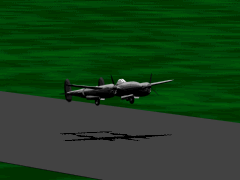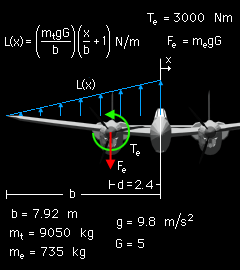Ch 8. Internal Loads Multimedia Engineering Statics Int. Forcesand Moments V and M Diagrams I V and M Diagrams II V, M, Load Relationships
 Chapter 1. Basics 2. Vectors 3. Forces 4. Moments 5. Rigid Bodies 6. Structures 7. Centroids/Inertia 8. Internal Loads 9. Friction 10. Work & Energy Appendix Basic Math Units Sections Search eBooks Dynamics Fluids Math Mechanics Statics Thermodynamics Author(s): Kurt Gramoll ©Kurt GramollSTATICS - CASE STUDY IntroductionProblem Graphic The wing of a P-38 is designed to withstand a 5g maneuver without damage. In order to design the structure, engineers needed to know the internal loads generated by the aerodynamic lift, and the mass and torque of the engine. What is known: The total airplane mass mt is 9,050 kg. The engine mass me is 735 kg. The engine torque Te is 3,000 Nm. The distance d from the centerline to the engine is 2.4 m. The span b of one wing is 7.92 m. The dynamic load factor G is 5. The acceleration due to gravity is 9.8 m/s2. The lift distribution is given by:     L(x) = (mt gG/b)(x/b+1) N/mForce Diagram Question What are the internal loads in the wing structure of the airplane during a 5g maneuver? Due to symmetry, only one wing needs to be calculated. Approach Develop shear and moment diagrams for the aerodynamic lift, engine mass, and engine torque separately using the relationships between load, shear, and moment. Using the principle of superposition, combine the three diagrams to get the shear and moment diagrams for the complete structure.

Practice Homework and Test problems now available in the 'Eng Statics' mobile app
Includes over 500 problems with complete detailed solutions.
Available now at the Google Play Store and Apple App Store.